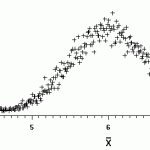# Satterthwaite Approximation

Probability and Statistics > T-Distributions > Satterthwaite approximation

Pooled standard error (how to find it).

## What is the Satterthwaite Approximation?

Watch the video for an overview and example of the formula:The Satterthwaite approximation is a way to account for two different sample variances. Basically, there are two ways to account for two sample variances:

1. Use the pooled standard error formula: Sp √ (1/n1 + 1/n2)
2. Use Satterthwaite’s: Se = √ (s12/n1 + s22/n2)

The two formulas are essentially equivalent — they will both give exactly the same answer. However, there are significant differences when the variances for the two samples are different. The pooled SE can only be used when your variances are equal — which almost never happens in real life. When your variances are not equal, use the Satterthwaite approximation. In fact, the Satterthwaite is always correct, so you may want to consider always using it over the pooled SE.

## Satterthwaite Approximation: Steps

Example problem: Use the Satterthwaite approximation for the following sample data:

• Sample 1: s = 20, n = 50.
• Sample 2: s = 15, n = 40.

Step 1: Insert your data into the formula. Note that:
n1 is the sample size from the first sample; n2 is the sample size from the second sample;
s1 is the standard deviation from the first sample; s12is the variance.
s2 is the standard deviation from the first sample; s22is the variance.

Se = √ (202/50 + 152/40)

Step 2: Solve:
Se = √ (8 + 5.625) ≈ 3.691

The Satterthwaite approximation is roughly equal to 3.691.

That’s it!

## References

Andreasen, C. & McDonald, A. (2021). 5 Steps to a 5: AP Statistics 2021 Elite Student Edition 1st Edition. McGraw-Hill Education.

CITE THIS AS:
Stephanie Glen. "Satterthwaite Approximation" From StatisticsHowTo.com: Elementary Statistics for the rest of us! https://www.statisticshowto.com/satterthwaite-approximation/
---------------------------------------------------------------------------Need help with a homework or test question? With Chegg Study, you can get step-by-step solutions to your questions from an expert in the field. Your first 30 minutes with a Chegg tutor is free!